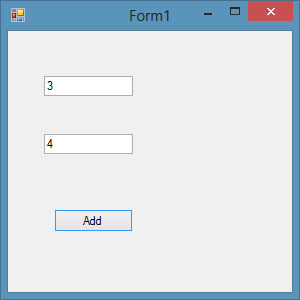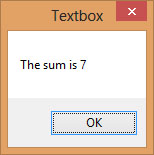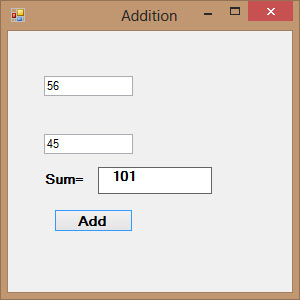# Visual Basic 2013 Lesson 5: Working with Controls

#### [Lesson 4] << [Contents] >> [Lesson 6]

In the previous lesson, you have learned how to write simple Visual Basic 2013 code. In this lesson, you will learn how to work with some common controls and write codes for them. Some of the common controls are the label, text box, button, list box and the combo box. However, in this lesson, you will only deal with the text box and the label, you shall learn how to work other controls in later lessons.

### 5.1 The TextBox

The textbox is the standard control for receiving input from the user. It can also be used to display the output. It can only handle string (text) and numeric data but not images or pictures. By the way, a string in a text box can be converted to a numeric data by using the function Val(text). The following example illustrates a simple program that processes the input from the user.

#### Example 5.1

In this program, you add two text boxes and a button on the form. The two text boxes are used to accept inputs from the user. Besides, a button is also programmed to calculate the sum of the two numbers using the plus operator. The value enters into a text box is stored using the syntax Textbox1.Text, where Text is one of the properties of the textbox.

The following program will add the value in text box 1 and value in text box 2 and output the sum in a message box.

```Private Sub Button1_Click(sender As Object, e As EventArgs) Handles Button1.Click
MsgBox(The sum is & Val(TextBox1.Text) + Val(TextBox2.Text))
End Sub
```

The output window:Figure 5.1

After clicking Add button, you can get the answer in a message box, as shown in Figure 5.2:Figure 5.2

### 5.2 The Label

The label is not only used to provide instructions and guides to the users, it can also be used to display outputs. It is different from text box because it can only display static text, which means the user cannot change the text. Using the syntax Label.Text, it can display text and numeric data. You can change its text in the properties window and also at runtime.

Example 5.2

Based on Example 5.1, you now add two labels, one is to display the text Sum= and the other label is to display the answer of the Sum. For the first label, change the text property of the label by typing Sum= over the default text Label1. Further, change its font to bold and font size to 10. For the second label, delete the default text Label2 and change its font to bold and font size to 10. Besides that, change its background color to white.

In this program, instead of showing the sum in a message box, we wish to display the sum on the label.

The Code

```Private Sub Button1_Click(sender As Object, e As EventArgs) Handles Button1.Click
Label2.Text = Val(TextBox1.Text) + Val(TextBox2.Text)
End Sub
```

*The function Val is to convert text to a numeric value. Without using Val, you will see that two numbers are joined together without adding them.

The output is as shown in Figure 5.3